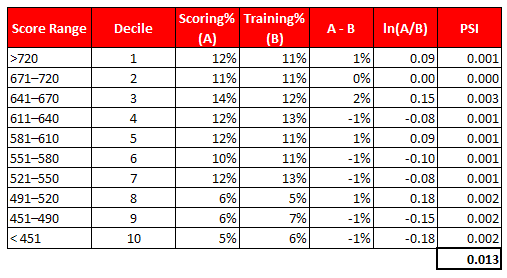# Population Stability Index and Characteristic Analysis

This tutorial describes the meaning and use of Population Stability Index and Characteristic Analysis.

In simple words, Population Stability Index (PSI) compares the distribution of a scoring variable (predicted probability) in scoring data set to a training data set that was used to develop the model. The idea is to check "How the current scoring is compared to the predicted probability from training data set".

## Use of Population Stability Index (PSI)

There are multiple uses of Population Stability Index (PSI). They are listed below -

1. Model might be influenced by economic changes. Suppose you built a risk model during economic recession (year 2008) and you are using the same model to score datasets in year 2016. There is a high chance that various attributes of the model are changed drastically over last 8 years. It means it does not make sense to use this model anymore if features of the model are changed significantly.
2. Change in product offerings due to internal policy changes. For example, one of your product are relaunched recently so attributes may behave differently as compared to attributes of your model.
3. PSI can detect if any data integration or programming issues to run the scoring code.

## How PSI is calculated?

PSI = (% of records based on scoring variable in Scoring Sample (A) - % of records based on scoring variable in Training Sample (B)) * In(A/ B)
Steps to calculate PSI
1. Sort scoring variable on descending order in scoring sample
2. Split the data into 10 or 20 groups (deciling)
3. Calculate % of records in each group based on scoring sample
4. Calculate % of records in each group based on training sample
5. Calculate difference between Step 3 and Step 4
6. Take Natural Log of (Step3 / Step4)
7. Multiply Step5 and Step6Population Stability Index
Rules : Population Stability Index (PSI)
1. PSI < 0.1 - No change. You can continue using existing model.
2. PSI >=0.1 but less than 0.2 - Slight change is required.
3. PSI >=0.2 - Significant change is required. Ideally, you should not use this model any more.
To understand the cause of a change, we need to generate the characteristic analysis report.

Note : We can use chi-square for binned data or KS test to compare distribution of two data sets.

## Characteristic Analysis

It answers which variable is causing a shift in population distribution. It compares the distribution of an independent variable in the scoring data set to a development data set. It detects shifts in the distributions of input variables that are submitted for scoring over time.

It helps to determine which changing variable is most influential in causing the model score shift.
Most Important Point-

Check the direction of impact due to model variable shifts.

Check the signs of the shifted attributes and the average values of those attributes compared to those from the previously scored population or development sample. This will indicate whether the model attribute shifts are increasing or decreasing the model scores.
Related Posts
ShareDeepanshu founded ListenData with a simple objective - Make analytics easy to understand and follow. He has over 10 years of experience in data science. During his tenure, he worked with global clients in various domains like Banking, Insurance, Private Equity, Telecom and HR.

15 Responses to "Population Stability Index and Characteristic Analysis"
1.2.Good article as well

3.4.Is there any rule for characteristic analysis just like mentioned in PSI.

5.Hello sir,
Very nicely explaned.. i am big friend of listendata.. I have 2 questions:
1) psi>.25 then what will be next step.. what changes we will do to bring it back to acceptable range..

2) If VSI> .25 then are we going to remove that variable

6.Hello,
Why here uses PSI>0,2.
value of 0.2 based on what?

1.if you see, PSI/CSI calculation is same as Information Value. so the thresholds can be understood the the same as Information value, PSI of 0.2 means an IV of 20. this suggests that the difference in population distribution across 2 vintages is as discriminatory as a variable with IV of 20%.

2.i didn't get it can you please elaborate it

7.HI Deepanshu,

I really love your blog.So is it possible for you to share a python script which calculate the population stability index(PSI) automatically.

8.Is it possible to calculate csi in python

9.Can you share CSI Code in Python?

10.is it possible to built psi without training data

11.so easy to understand and informative!

12.I was trying to use the PSI on the binary variables(0, 1), but it's not working as expected.
I have below PSI on binary variables(bin 1: value 1, bin 2: value 0), bin 1 and 2 changed significantly. But the PSI value is very less.

bin Expected Actual % Expected % Actual PSI
1 25,034 7,223 0.0321052 0.0127180 0.0179527
2 754,714 560,714 0.9678948 0.9872820 0.0003845
Total 779,748 567,937 1.0000000 1.0000000 0.0183372

13.Could you suggest how to find an optimal size of the data for which we want to measure PSI w.r.t. training set?

Next → ← Prev
Looks like you are using an ad blocker!

To continue reading you need to turnoff adblocker and refresh the page. We rely on advertising to help fund our site. Please whitelist us if you enjoy our content.